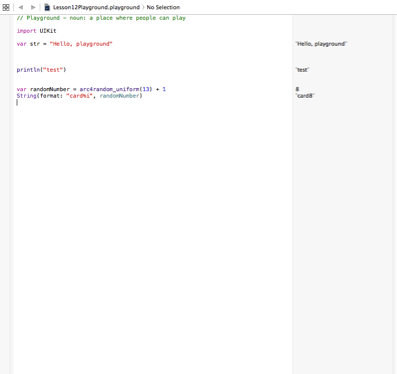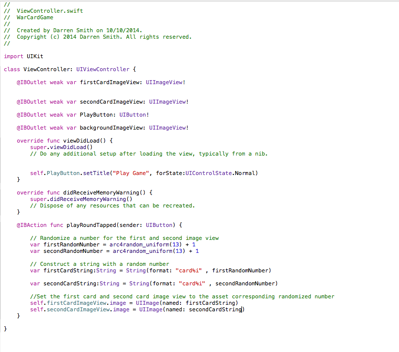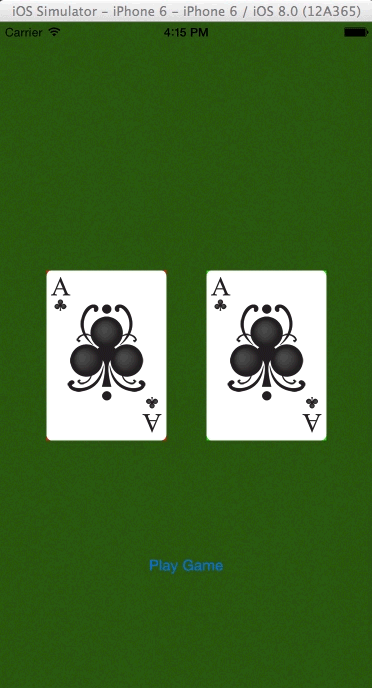# Xcode Swift Code: Randomising the Cards for the Card Game

Randomising the Cards for the Card Game

Today I learned how to produce random numbers and change the card images when the button is pressed.
In order to learn how created random numbers, I went back to Xcode playground. In order to generate a number I used arc4random_uniform() function. What this function does is it will look for a number between ranges of 0 - the enter you give it. Below in figure 1 is an illustration of the function.Figure 1: Using Xcode Playground to experiment the random number Class

Now that I understand how the function works, i then headed back to the card game project in Xcode 6. I then applied the arc4random() function for the UI Image card image underneath the IB Action button action function. Below in figure 2 is the implementation of the function to the game.Figure 2: Implementing the random class to the Card game, so random cards will be revealed when the user presses the play button.

Once the code is implemented, the application is tested to see if random cards are revealed each time the user presses the play button. The result is shown below in figure 3.Figure 3: The End result